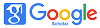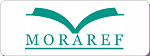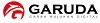### PENGARUH RETURN ON ASSET DAN RETURN ON EQUITY TERHADAP PRICE EARNING RATIO PADA PERUSAHAAN YANG TERDAFTAR DI JII

#### Abstract

Investors tend to calculate the company's ability to manage profits from assets and capital owned will affect the earnings value reflected in the price of a company's stock. Price Earning Ratio (PER) or also called earnings multiplier approach is a popular approach that uses earnings value to estimate intrinsic value. The data analysis technique used is multiple linear regression data analysis using spss 21 software. With companies listed on The Jakarta Islamic Index as a research sample shows that the ROA variable has a significant effect on PER seen from the t test where t counts 5.179> t table 1.658 with a significance of 0.000 <0.05 and with a coefficient value of 0.875. ROE variable has a significant effect on PER seen from t test where t counts 4.191> t table 1.658 with a significance of 0.000 <0.05 and with a coefficient value of 0.716. Simultaneously the ROA and ROE variables have a significant effect on PER with the value of Fcount 72.260> Ftable 3.08 with a sig value of 0.000 <0.05

#### Keywords

On Asset, Return On Equity, Price Earning Ratio

PDF

### Refbacks

• There are currently no refbacks.